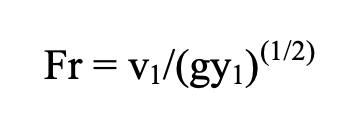# Applying the Froude Number to the Grande Ronde

Author
Ann Fissekis

The Froude number (Fr) is a dimensionless variable (i.e. it has no units) that describes the ration of inertia to gravitational forces on flow. It is a function of gravity, a fluid’s average velocity and depth.When Fr<1, gravity is the dominant force driving the fluid’s motion; flow is called subcritical. When Fr>1, inertia is the driving force; flow is called supercritical. Critical flow occurs when inertial and gravitational forces are balanced and Fr=1.

On the Wenaha River, a 5th order tributary to the Grande Ronde River, average velocity and depth was measured across a relatively uniform cross-section. The results are displayed below:

Table 1 Froude number calculations for a relatively uniform cross-section

 Average velocity (m/s) Depth (m) Fr 0.5 0.7 0.19 - subcritical

The Froude number is less than one, indicating that the flow in the cross-section is subcritical. This is consistent with the observed properties of the flow: the area was characterized as a pool (see Figure 1) with a relatively low gradient. Those qualities indicate relatively low velocities, which are characteristic of subcritical flows.Figure 1. Wenaha River pool within which the velocity/depth profile was conducted
Associated File(s)